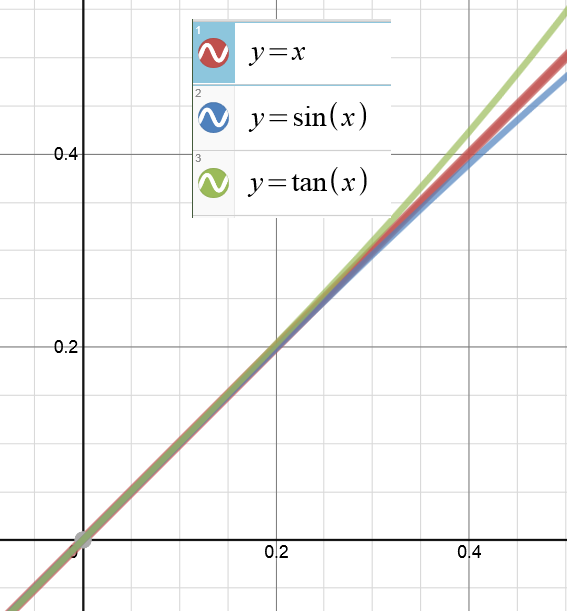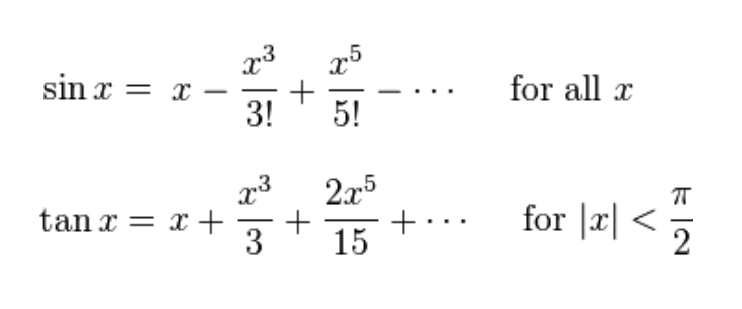# For Small Angles Only

Two Surprising Trigonometric Almost-Equalities

Let’s warm up with an old math joke I first heard back in my own freshman year.  I have always thought it was funny even though I am not sure I get it.  But my students all LOVE my math jokes, so here goes:I think it is meant to make fun of the way we sometimes do math in physics. For example…

When we are studying Young’s two-slit interference experiment, there comes a point when my students are asked to accept that for small angles, two things that they know are NOT equal can be sort-of, kind-of treated as equal:

sin(θ) ≈ tan(θ)

And when we are studying simple harmonic motion, I ask them to accept another one:

sin(θ) ≈ θ

That one is also only “true” when the small angles are measured in RADIANS (as you will see if you bravely read on).

Once you accept these two things, you can go on to derive other interesting rules about interference patterns and about the behavior of pendulums.  But why should anyone accept these things?  I’m going to give you a collection of reasons.

The chart above shows you values of the angle in degrees and then the sine, the radian value and the tangent listed in that order.  You can see that the values are close to each other.  The sine is a little less than the radian measure and the tangent is a little greater.  But even for angles as big as 20 degrees, the differences are less than 5%.  So just looking at the numbers, you have to accept that for small angles, these three functions — sin(θ), tan(θ) and θ — are equal-ish.

2. Look at the Graphs

Here you see the graphs of the three functions graphed using the Desmos website:OK, clearly these are three very different functions.  But we are not interested in their entire domains.  We have only claimed that the functions are nearly equal for “small” angles.  So let’s just zoom in on the section of the graph from 0 to .4 radians…Again, if we stay where the angles are small, the three graphs are “equal-ish.”

3. Look at their Taylor Series Representations

This is something that students learn later in their first year of calculus.  These non-polynomial functions can each be expressed as a polynomials with an infinite number of terms.  And they can be approximated with polynomials of finite length.

How these polynomial formulas are developed and how much error you introduce by using only a finite number of terms are topics for several weeks of discussion in calc class.  But for now, let’s look at the first few terms of the Taylor polynomials for the sine function and the tangent function:Notice that the first term is the same: it’s just ‘x’.  Then, after that, the next term has x-cubed.  But if x is already small, then x-cubed is going to be REALLY small.  Negligibly small.  As we already expect from looking at the charts and graphs, sin(x) is a little bit smaller than x and then tan(x) is a little bigger than x.  But for small values, the differences are negligible.

I know you are probably already convinced. But I have one more reason for you to believe this and it gives me an excuse to re-use one of my favorite diagrams…

4. Look at the Geometry

Here it is again:This was the diagram we used to learn the geometric meaning of all of the trig ratios.  Now I am going to make some changes to the diagram:  I am going to make the angle smaller and also I am going to delete any of the segments we don’t need right now.  Then I am going to zoom in on the part we care about:The length of the blue segment is sin(θ).  The length of the orange segment is tan(θ).  And because it is a unit circle, the length of that intercepted arc is just θ.  (If you are not sure why that is true, think about the definition of radian measure — or read about it in this earlier post here.)

Now look at how the lengths of those two segments compare to the length of the arc.  One of them (the sine) is a little shorter.  One of them (the tangent) is a little longer.  But as the angle gets smaller and smaller, the differences become negligible!

Well, I hope one of these four methods have convinced you that this is legal.  If not, I hope at least that you enjoyed the joke at the beginning.